# Rectangle - Definition with Examples

The Complete K-5 Math Learning Program Built for Your Child

• 40 Million Kids

Loved by kids and parent worldwide

• 50,000 Schools

Trusted by teachers across schools

• Comprehensive Curriculum

Aligned to Common Core

## What is Rectangle?

A rectangle is a 2D shape in geometry, having 4 sides and 4 corners. Its two sides meet at right angles. Thus, a rectangle has 4 angles, each measuring 90 ̊. The opposite sides of a rectangle have the same lengths and are parallel.  Two sides are said to be parallel, when the distance between them remains the same at all points.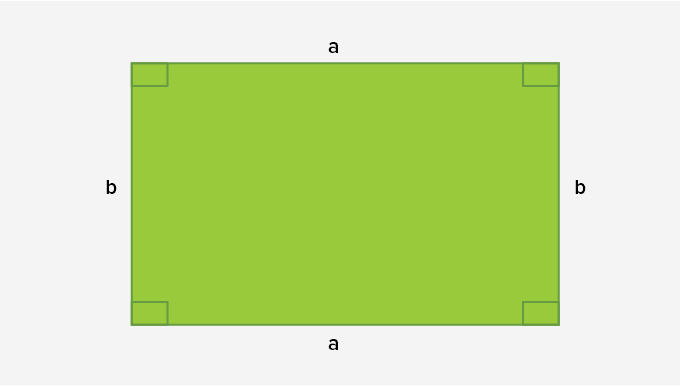Other names of the rectangle

• Since all the angles of a rectangle are equal, we also call it an equiangular quadrilateral.

• Since it has parallel sides, we can also call it a parallelogram. A parallelogram is a quadrilateral whose opposite sides are equal and parallel.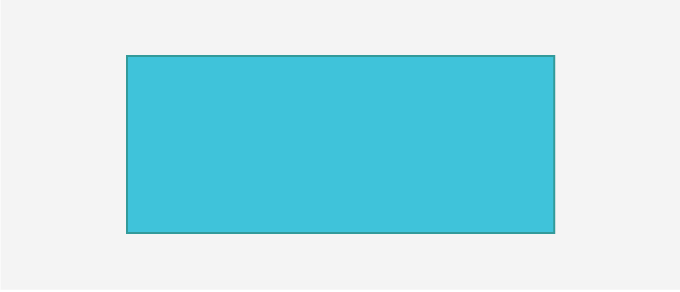Parallelogram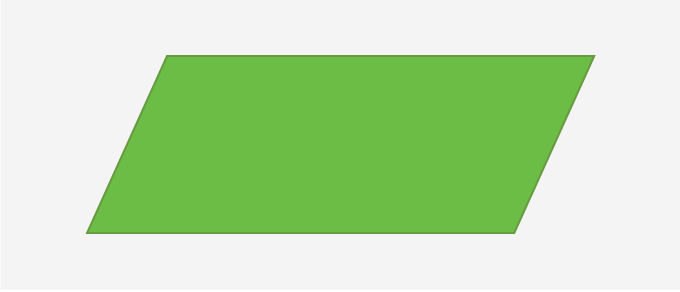## Properties of a rectangle:

• It is a flat shape

• It has 4 sides (edges)

• It has 4 corners (vertices)

• It has 4 right angles

## Area of a rectangle:

The area of a rectangle is the product of its two adjacent sides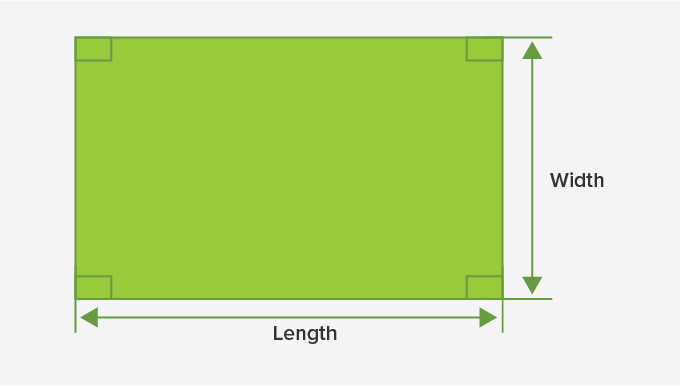Area of a rectangle = Length × Width

Perimeter:

The perimeter of a rectangle is the sum of the length of its four sides.  Since parallel sides of a rectangle have the same length, the formula for the perimeter of a rectangle is

Perimeter of a rectangle = Length + Width + Length + Width

2 length + 2Width

2 (length + Width)

Diagonals of a rectangle:

The line segments that join the opposite corners of a rectangle are called its diagonals.  In the given figure, the two diagonals of the rectangle are AC and BD. The diagonals of a rectangle are the same in length.  Therefore, AC = BD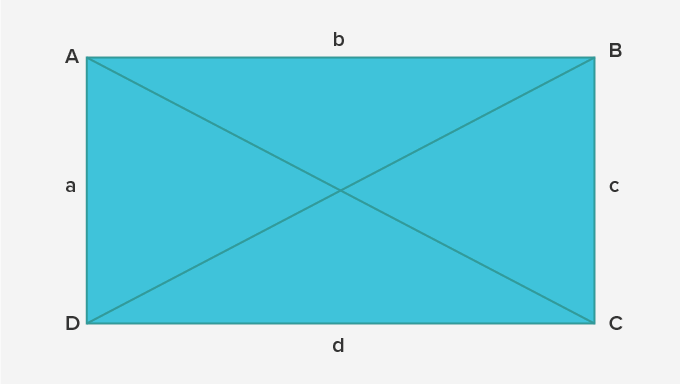Rectangle being the most common shape forms a part of our day to day life.  Some real-life examples of the rectangle are table tops, books, mobile phones, TV, etc.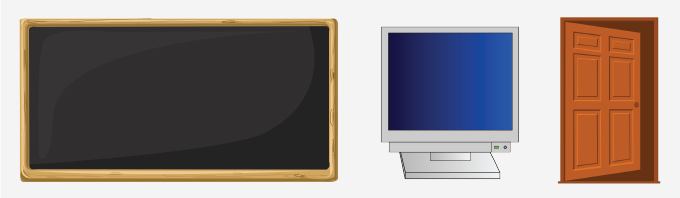Fun Facts All rectangles are parallelograms, but all parallelograms are not rectangles The diagonals of a rectangle divide the rectangle into four triangles Every square is a rectangle, but every rectangle is not a square

Won Numerous Awards & Honors